Remarking¶

Got something to highlight? Make a remark!

trigger condition¶

To add a remark, pass a condition; upon meeting that condition, the remarks will appear.

:
import numpy as np
import ahlive as ah
xs = np.array([0, 1, 2])
ys = np.array([3, 4, 5])
arr = ah.Array(xs, ys)
arr = arr.remark(
condition=ys == 4,
remarks='y equals to 4!!'
)
arr.render()
: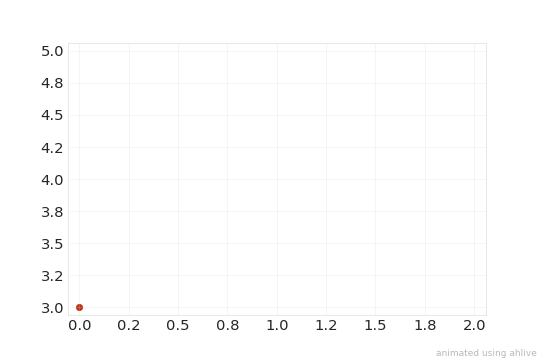remark does not save the object inplace so be sure to either save it to a new variable or same variable!

Sometimes, when joining ah.Arrays, using condition can get flaky.

:
import numpy as np
import ahlive as ah

xs = np.array([0, 1, 2])
ys = np.array([3, 4, 5])

arr = ah.Array(xs, ys)
arr = arr - arr

try:
arr = arr.remark(
condition=ys == 4,
remarks='y equals to 4!!'
)
arr.render()
except ValueError as e:
print(e)
operands could not be broadcast together with shapes (3,) () (2,6)

To get around that, use the xr.Dataset under data for condition.

:
import numpy as np
import ahlive as ah

xs = np.array([0, 1, 2])
ys = np.array([3, 4, 5])

arr = ah.Array(xs, ys)
arr = arr - arr

ds = arr.data[1, 1]

arr = arr.remark(
condition=ds["y"] == 4,
remarks='y equals to 4!!'
)
arr.render()
: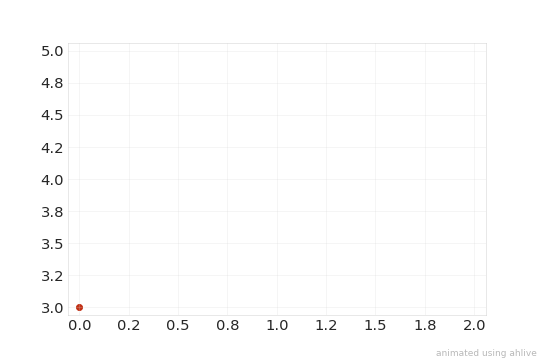:
import numpy as np
import ahlive as ah

xs = np.array([0, 1, 2])
ys = np.array([3, 4, 5])

arr = ah.Array(xs, ys)
arr = arr - arr

arr.reference(y0s="y", last=True).render()
: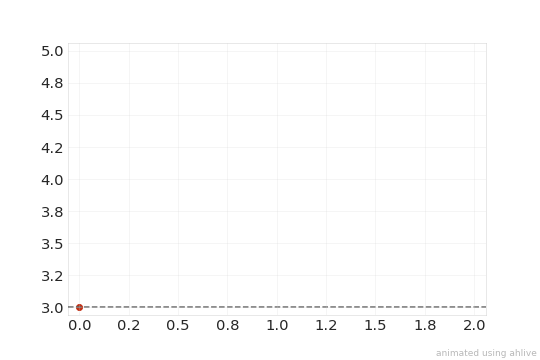convenient conditions¶

Instead of formulating a condition, for convenience, values can be passed to xs, ys, cs, labels, state_labels, and inline_labels.

When the data values match the conditional values for the given label, remarks will trigger.

:
import numpy as np
import ahlive as ah

xs = np.array([0, 1, 2])
ys = np.array([3, 4, 5])

arr = ah.Array(xs, ys)
arr = arr - arr

ds = arr.data[1, 1]

arr = arr.remark(
ys=4,
remarks='y equals to 4!!'
)
arr.render()
:Values to match can be Iterables as well.

:
import ahlive as ah
arr = ah.Array([0, 1, 2], [3, 4, 5])
arr = arr.remark(xs=[1, 2], remarks="y is matched!")
arr.render()
: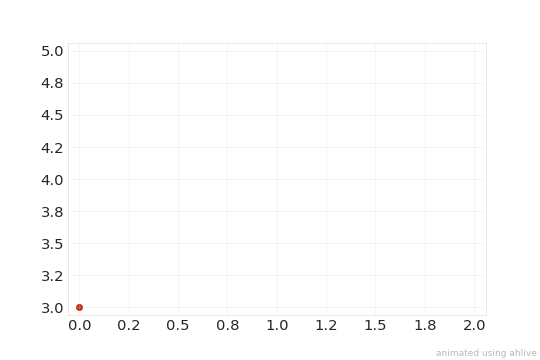Multiple remarks can be passed too, as long as it matches the number of states.

:
import ahlive as ah
arr = ah.Array([0, 1, 2], [3, 4, 5])
arr = arr.remark(xs=[1, 2], remarks=['wont show', '1st show', '2nd show'])
arr.render()
: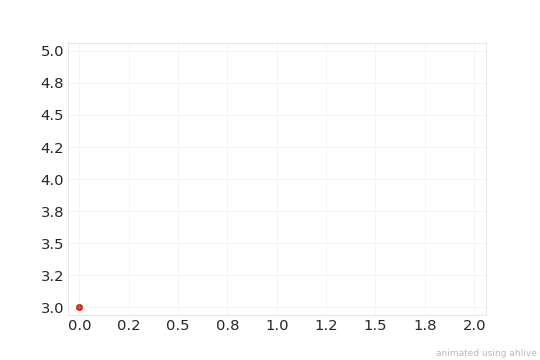Any labels listed under “Data variables” is valid for use as long as it contains a state dimension.

:
import ahlive as ah
arr = ah.Array([0, 1, 2], [3, 4, 5], s=[6, 7, 8])
print(arr)
arr = arr.remark(s=[6, 7], remarks=['the size is 6', 'the size is 7', ''])
arr.render()
<ahlive.Data>
Subplot:         (1, 1)
Dimensions:      (item: 1, state: 3)
Data variables:
x        (item, state) float64 0.0 1.0 2.0
y        (item, state) float64 3.0 4.0 5.0
s        (item, state) float64 6.0 7.0 8.0
chart    (item) <U7 'scatter'
label    (item, state) <U1 '' '' ''
group    (item) <U1 ''
Attributes (0/49):

: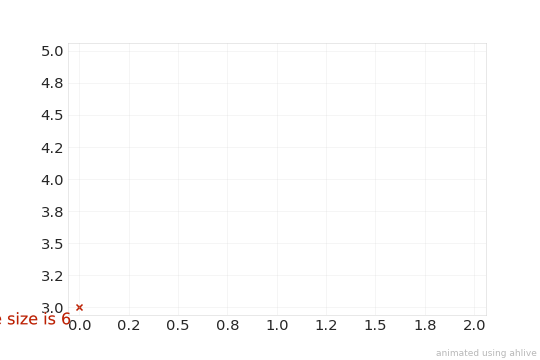dynamic remarks¶

Rather than setting static values for remarks, passing a label from the dataset, e.g. x, y, c, label, state_label, and inline_label (without the “s” suffix), can dynamically grab the value for that label at that given condition. Any labels listed under “Data variables” is valid for use as long as it contains a state dimension.

:
import ahlive as ah
arr = ah.Array([0, 1, 2], [3, 4, 5])
print(arr)
arr = arr.remark(xs=[1, 2], remarks='x')
arr.render()
<ahlive.Data>
Subplot:         (1, 1)
Dimensions:      (item: 1, state: 3)
Data variables:
x        (item, state) float64 0.0 1.0 2.0
y        (item, state) float64 3.0 4.0 5.0
chart    (item) <U7 'scatter'
label    (item, state) <U1 '' '' ''
group    (item) <U1 ''
Attributes (0/49):

: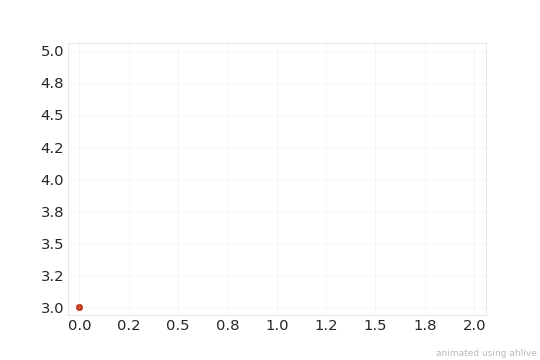Note, remarks will be triggered every time the conditional value is met.

:
import ahlive as ah
arr = ah.Array([0, 1, 2], [3, 4, 4])
arr = arr.remark(ys=4, remarks='y')
arr.render()
: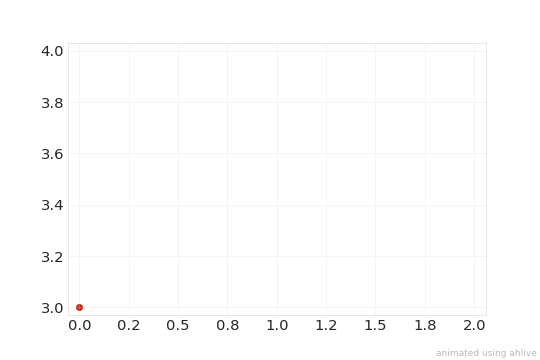delay durations¶

It is also possible to add delays, in seconds, where there was a remark through durations.

:
import numpy as np
import ahlive as ah
xs = np.array([0, 1, 2])
ys = np.array([3, 4, 5])
arr = ah.Array(xs, ys)
arr = arr.remark(
condition=ys == 4,
remarks='y equals to 4!!',
durations=2
)
arr.render()
:durations is only supported with gif, i.e. not video formats!

first encounter¶

To have the remarks trigger only once on the initial match, set first to True.

:
import ahlive as ah
arr = ah.Array([0, 1, 2], [3, 4, 4])
arr = arr.remark(ys=4, remarks='y', first=True)
arr.render()
gifsicle: warning: trivial adaptive palette (only 255 colors in source)
: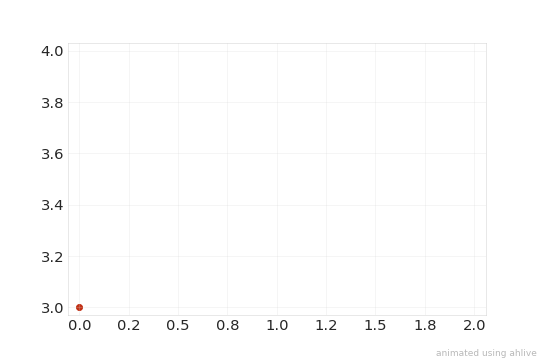first operates separately on each conditional value, i.e. remarks will trigger for 3 and the first 4, but not the last 4.

:
import ahlive as ah
arr = ah.Array([0, 1, 2], [3, 4, 4])
arr = arr.remark(ys=[3, 4], remarks='y', first=True)
arr.render()
: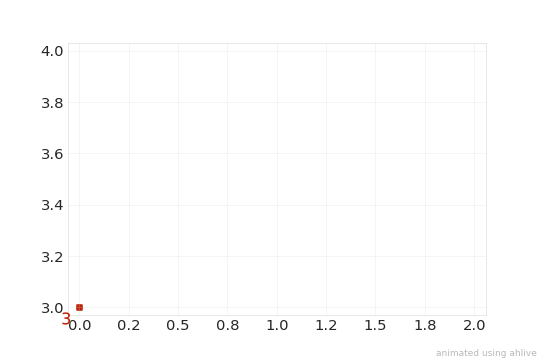first does not work with manually input condition, only with convenient conditions.

persist plot¶

To have the plot marker persist:

:
import ahlive as ah
arr = ah.Array([0, 1, 2], [3, 4, 4])
arr = arr.remark(ys=4, remarks='y', persist_plot=True)
arr.render()
:persist inline¶

To have the inline label persist:

:
import ahlive as ah
arr = ah.Array([0, 1, 2], [3, 4, 4])
arr = arr.remark(ys=4, remarks='y', persist_inline=True)
arr.render()
: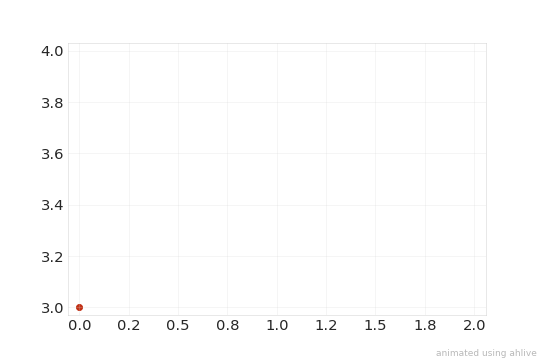tolerance levels¶

An absolute tolerance atol can be specified for inexact matching.

:
import ahlive as ah
arr = ah.Array([0, 1, 2], [3, 4, 4])
arr = arr.remark(ys=4.15, remarks='y', atol=0.5)
arr.render()
:A relative tolerance rtol can be passed too.

:
import ahlive as ah
arr = ah.Array([0, 1, 2], [3, 4, 4])
arr = arr.remark(ys=4.15, remarks='y', rtol=0.1)
arr.render()
:rtol and atol cannot be used with the condition keyword.